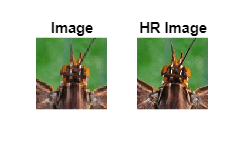# Deploy Super Resolution Application That Uses TensorFlow Lite (TFLite) Model on Host and Raspberry Pi

This example shows simulation and code generation for a super resolution application by using a Tensorflow Lite model. The task of recovering a high resolution (HR) image from its low resolution counterpart is commonly referred to as Single Image Super Resolution (SISR). In this example, you use TFLite to run inference on a pretrained ESRGAN (ESRGAN: Enhanced Super-Resolution Generative Adversarial Networks) model.

The TFLite model used in this example upsamples a 50x50 low resolution image to a 200x200 high resolution image (scale factor=4).

This example is supported for host Windows® and Linux® platforms.

This example uses TFLite to run inference on a pretrained ESRGAN model.

Run these commands to download the ESRGAN network from the URL mentioned below.

```if ~exist("esrgan-tf2.tflite","file") disp('Downloading 5 MB ESRGAN model file...'); url = "https://tfhub.dev/captain-pool/lite-model/esrgan-tf2/1?lite-format=tflite"; websave("esrgan-tf2.tflite",url); end```

You can use any other super resolution model test this workflow by following the steps mentioned below. If you have only a TensorFlow model for your network, use these steps to convert the TensorFlow model to a TFLite model.

Run these commands to download the image from the URL mentioned below.

```if ~exist("inputsuperresolution","file") disp('Downloading input file...'); url = "https://raw.githubusercontent.com/tensorflow/examples/master/lite/examples/super_resolution/android/app/src/main/assets/lr-1.jpg"; websave("inputsuperresolution.jpg",url); end```
```Downloading input file... ```

Read the input image and display it.

```I = imread('inputsuperresolution.jpg'); imshow(I); title('Original Image');```### The `super_resolution_predict` Entry-Point Function

In this example, you generate code for the entry-point function `super_resolution_predict.m`. This function loads the ESRGAN model into a persistent network object by using the `loadTFLiteModel` function. Then the function performs prediction by passing the network object to the `predict` function. Subsequent calls to this function reuse this persistent object.

`type super_resolution_predict.m`
```function out = super_resolution_predict(in) persistent net; if isempty(net) net = loadTFLiteModel('esrgan-tf2.tflite'); net.Mean = 0; net.StandardDeviation = 1; % Set number of threads based on max number of threads in host / hardware % net.NumThreads = 4; end out = net.predict(in); %% Post processing Output % Cast output to uint8 out = uint8(out); end ```

The `loadTFLiteModel` function creates a `TFLiteModel` object. The properties of this object contain information about the model such as the number and size of inputs and outputs of the model.

```net = loadTFLiteModel('esrgan-tf2.tflite'); % Display Input Size disp(net.InputSize);```
``` {[50 50 3]} ```
```% Display Output Size disp(net.OutputSize); ```
``` {[200 200 3]} ```

### Resize Image Based on TFLite Model Input Shape

Use `imresize` to resize the input image based on TFLite model input shape.

`I = imresize(I,net.InputSize{1}(1:2));`

To perform inference by using the loaded TFLite model, use any of the following workflows.

### Workflow 1: Perform Super Resolution by Using Simulation on Host

Run the simulation by passing the input image `I` to the entry-point function.

`predictionScores = super_resolution_predict(I);`
``` TFLiteModel with properties: ModelName: 'esrgan-tf2.tflite' NumInputs: 1 NumOutputs: 1 InputSize: {[50 50 3]} OutputSize: {[200 200 3]} InputScale: 1 InputZeroPoint: 0 OutputScale: 1 OutputZeroPoint: 0 InputType: {["FP32"]} OutputType: {["FP32"]} NumThreads: 6 Mean: 0 StandardDeviation: 1 ```

### Workflow 2: Perform Super Resolution by Running Generated MEX on Host

• MATLAB® Coder™

This example uses the `codegen` (MATLAB Coder) command to generate a MEX function that runs on the host platform.

#### Generate MEX Function

To generate a MEX function for a specified entry-point function, create a code configuration object for a MEX. Set the target language to C++.

```cfg = coder.config('mex'); cfg.TargetLang = 'C++';```

Run the `codegen` command to generate the MEX function `super_resolution_predict_mex` on the host platform.

```codegen -config cfg super_resolution_predict -args ones(50,50,3,'uint8') ```

#### Run Generated MEX

Run the generated MEX by passing the input image `I`.

```predictionScores = super_resolution_predict_mex(I); ```

### Workflow 3: Generate Code for Super Resolution Application, Deploy and Run on Raspberry Pi

• MATLAB® Coder™

• Embedded® Coder™

• MATLAB Support Package for Raspberry Pi Hardware. To install this support package, use the Add-On Explorer.

#### Third-Party Prerequisites

• Raspberry Pi hardware

• TFLite library (on the target ARM® hardware)

On the Raspberry Pi hardware, set the environment variable `TFLITE_PATH` to the location of the TFLite library. For more information on how to build the TFLite library and set the environment variables, see Prerequisites for Deep Learning with TensorFlow Lite Models.

#### Set Up Connection with Raspberry Pi

Use the MATLAB Support Package for Raspberry Pi Hardware function `raspi` to create a connection to the Raspberry Pi.

In the following code, replace:

• raspiname with the name of your Raspberry Pi board

```r = raspi('raspiname','username','password'); ```

#### Copy TFLite model to Target Hardware

Copy the TFLite model to the Raspberry Pi board. On the hardware board, set the environment variable `TFLITE_MODEL_PATH` to the the location of the TFLite model. For more information on setting environment variables, see Prerequisites for Deep Learning with TensorFlow Lite Models.

In the following commands, replace:

• `targetDir` with the destination folder of TFLite model on the Raspberry Pi board.

```r.putFile('esrgan-tf2.tflite',targetDir) ```

#### Generate PIL MEX Function

To generate a PIL MEX function for a specified entry-point function, create a code configuration object for a static library and set the verification mode to `'PIL'`. Set the target language to C++.

```cfg = coder.config('lib','ecoder',true); cfg.TargetLang = 'C++'; cfg.VerificationMode = 'PIL';```

Create a `coder.hardware` (MATLAB Coder) object for Raspberry Pi and attach it to the code generation configuration object.

```hw = coder.hardware('Raspberry Pi'); cfg.Hardware = hw;```

On the host platform, run the `codegen` command to generate a PIL MEX function `super_resolution_predict_pil`.

```codegen -config cfg super_resolution_predict -args ones(50,50,3,'uint8') ```

#### Run Generated PIL MEX

Run the generated PIL executable by passing the input image `I`.

```predictionScores = super_resolution_predict_pil(I); ```

### Display the Super Resolution Image

```subplot(1,2,1); imshow(I); title('Image'); subplot(1,2,2); imshow(predictionScores); title('HR Image');```